Function Repository Resource:

# ErikssonCornerAngle

Evaluate the multidimensional corner angle

Contributed by: Michael Reed
 ResourceFunction["ErikssonCornerAngle"][o,{p1,…,pd}] gives the corner angle at the point o and spanned by the vectors p1,…,pd.

## Details

ResourceFunction["ErikssonCornerAngle"] generalizes the law of sines for d-dimensional Euclidean simplices.

## Examples

### Basic Examples (2)

The corner angle at the origin for vectors in two dimensions:

 In:=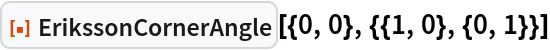Out=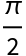The corner angle at the origin for vectors in three dimensions:

 In:=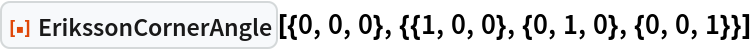Out=### Scope (1)

ErikssonCornerAngle works in any number of dimensions:

 In:=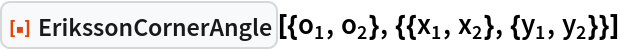Out=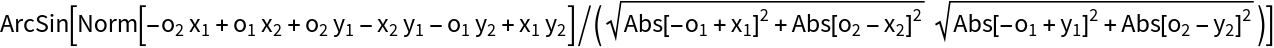In:=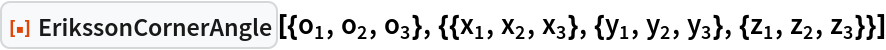Out=In:=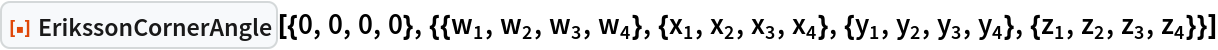Out=### Applications (2)

Compute the corner angle for various Platonic solids:

 In:=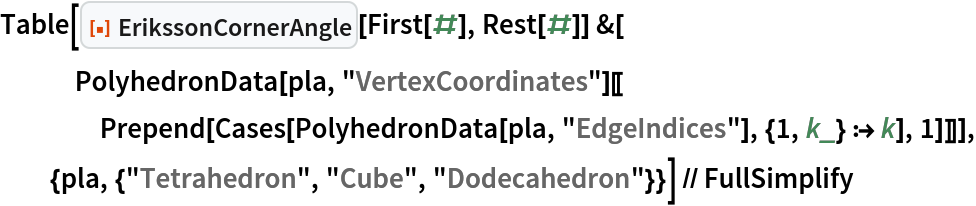Out=Generate a random simplex:

 In:=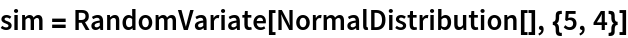Out=Compute all the corner angles of the simplex:

 In:=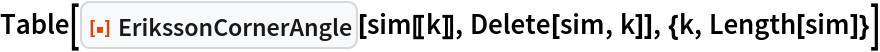Out=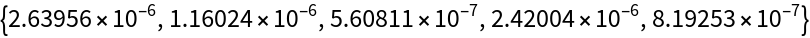### Properties and Relations (2)

In two dimensions, ErikssonCornerAngle is similar to the results of SolidAngle and PlanarAngle:

 In:=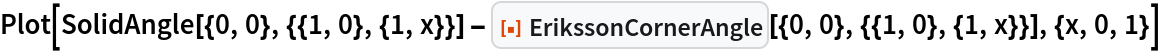Out=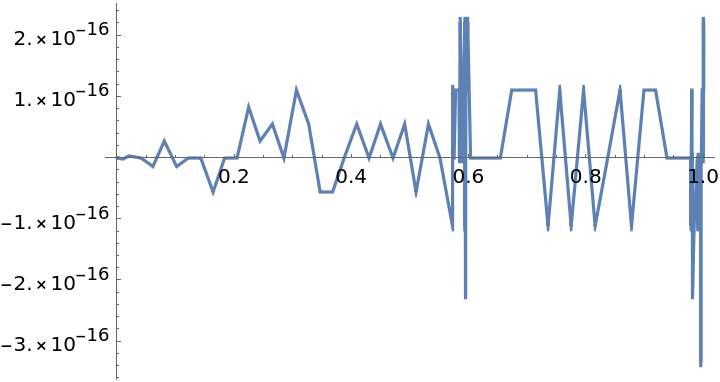In three dimensions, the difference between ErikssonCornerAngle and SolidAngle is more apparent:

 In:=Out=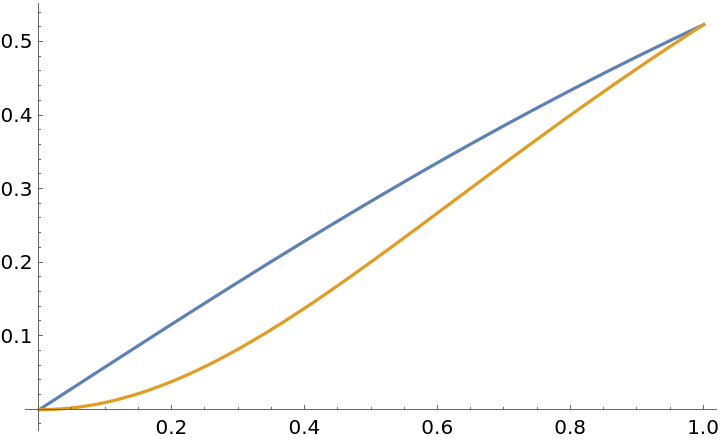Michael Reed

## Version History

• 1.0.0 – 20 July 2022